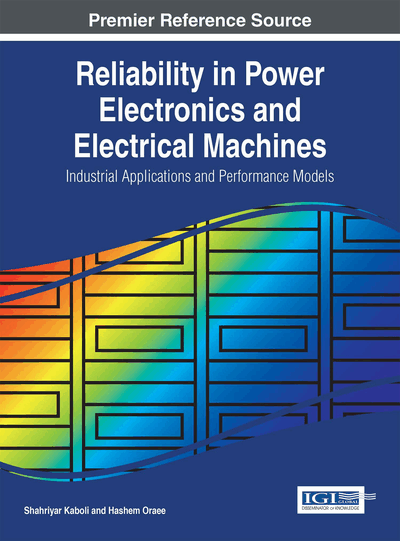# Reliability Measurement

DOI: 10.4018/978-1-4666-9429-3.ch005

## Abstract

After calculation of reliability, the system is constructed. It is important to measure the calculated useful life of the system. Reliability measurement tests can also be used for the converter operating in service to estimate the remaining useful life of the converter. In this chapter, various methods of tests for this goal are presented. The main approach is accelerated aging test that reduce the time needed for failure in a system. In this method, the device is tested under conditions beyond its defined nominal specifications. Limits for this harsh condition is determined based on the calculations which are presented in chapters 3 and 4. If a problem occurs in implementing and operating process of the converter, accelerated aging tests decrease the time to failure. Theoretical concept of accelerated aging tests is described. Standard tests of electric power converters are presented. Equipment and test chambers for standard tests are explained. These tests contain all four various failure factors which are presented in chapter 2. Sample industrial examples are presented to demonstrate the procedure of the tests. Some accelerated aging tests may lead to destruction of the converter. Difference between destructive and nondestructive tests is presented. Sample devices after accelerated aging tests are shown. Measuring devices for system parameter identification are introduced. Various types of tests are expressed in details for some of the most important tests like electric withstand tests.
Chapter Preview
Top

## Introduction

In Chapter 2, we reviewed the most important failure factors of an electric power converter. However, there are still many unknown failure factors that they become important in special cases. For example, one of the loss factors in electrical machines was stray load loss. This is an unknown factor and cannot be modeled precisely. In many cases, it is a small percent of failure factors. However, it may be dominant factor for the motors operating in special conditions. Theoretical analysis cannot consider all factors about reliability of a converter (Lu, & Rudy, 2001). Therefore, we need to test the reliability of a converter after implementation and before popular allocation. We study the commonly used methods for reliability testing in the current chapter. Figure 1 shows the state of this chapter in the flowchart of the book. But, here is a problem. Assuming an exponential distribution, a reasonable assumption for electronic components, the specific prediction for the product is simply a matter of determining operating hours and types of failures expected. The failure rate of the product can be determined from the following equation:

Failure Rate = Number of Failures / Operating Time

The advantage of predicting from field and test data is that the reliability results can be accurately determined including the associated uncertainty of the estimate (Wong, Albrecht, Allan, Billinton, Chen, Fong, Haddad, Li, Mukerji, Patton, Schneider, Shahidehpour, & Singh, 1999). The disadvantage is the difficulty of obtaining and assessing accurate field and test data. In addition, more important problem is the time interval that is necessary for fault occurrence.

Top

## Effect Of Test On Equipment

In reliability tests, at each test one or some parameters of environmental conditions are stressed more than typical state to reduction of test time less than real state. Then, there are some determined relations between this accelerated test results and typical condition results that are used to finding failure rates and reliability evaluation. One of the main concerns in these tests is this question: Does the over stress test have improper effect on the equipment? This question become more important when we want to evaluate the reminder useful life of a converter (Saha, 2003). In this case, the reliability tests must have no destructive effect on the equipment.

### Destructive Tests

In destructive testing, tests are carried out to the specimen's failure, in order to understand a specimen's structural performance or material behaviour under different loads. These tests are generally much easier to carry out, yield more information, and are easier to interpret than nondestructive testing. Destructive testing is most suitable, and economic, for objects which will be mass-produced, as the cost of destroying a small number of specimens is negligible 2.

## Complete Chapter List

Search this Book:
Reset